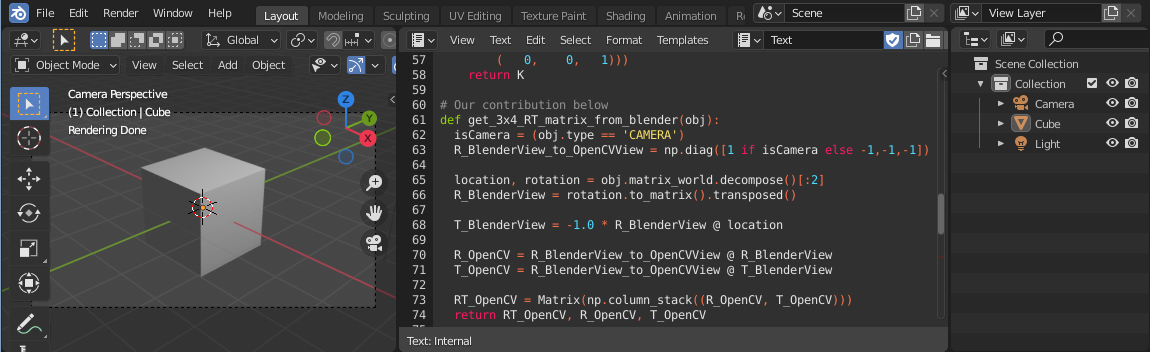In our previous post about computer vision, we showed our pipeline to solve a specific problem, and it involved some steps inside Blender and others on OpenCV. Also, I mentioned that it was not something straightforward to go from one to the other. Here we will describe how we transposed camera and objects from a scene from Blender to OpenCV.# Coordinate Systems

Blender has five documented coordinate orientations, but the two important here are Global and View orientations. OpenCV’s documentation describes both here.

Blender Global Blender View OpenCV Global OpenCV View
X to the view left to right left to right left to right
Y left to right down to up up to down up to down
Z down to up to the view to the view from the view

# The transformation from Blender to OpenCV

To transform from the Blender View orientation to the OpenCV View orientation, we need to keep X while inverting Y and Z signals. But there is a trick here: if we want to describe an object in front of the camera, we will need to invert X’s signal too, so we will use (X, -Y, -Z) if the object is the camera, but for another type, its (-X, -Y, -Z).

# Using the transformation

First, we can’t use Euler angles because of the same mathematical principle that causes “Gimbal Lock” (read here). So we will use the rotation-translation matrix.

Among other representations like quaternions, we choose to use the rotation-translation matrix to represent the pose of the object/camera. It is defined by a rotation matrix `R` and a translation vector `T`, both concatenated in a form of a `[R|T]` matrix.

For cameras, if the `[R|T]` matrix is further multiplied by the intrinsic parameters, we call it a projection matrix, so it feels very convenient and compact form to organize the information.

Before we jump into the implementation, as a reference, we would like to point this excellent answer from the StackOverflow community. Despite we didn’t need to do much to use that code to transpose the camera, we would like to describe the steps used, along with our small modification to transpose objects that are not a camera.

1. To satisfy both camera and other objects, the transformation matrix from Blender to OpenCV is modified accordinly:
``````R_BlenderView_to_OpenCVView = np.diag([1 if isCamera else -1,-1,-1])
``````
2. From Blender’s API, we can get the world transformation matrix for a given object calling `bpy.types.Object.matrix_world()`, then we can use `mathutils.Matrix.decompose()` to decompose it in terms of a location `Vector` and rotation `Quaternion`.
``````location, rotation = obj.matrix_world.decompose()[0:2]
``````
3. Transform from Blender Global orientation to Blender View orientation by transposing:
``````R_BlenderView = rotation.to_matrix().transposed()
``````
4. Apply Blender View rotation to the location:
``````T_BlenderView = -1.0 * R_BlenderView @ location
``````
5. Transform from Blender View orientation to OpenCV View orientation:
``````R_OpenCV = R_BlenderView_to_OpenCVView @ R_BlenderView
T_OpenCV = R_BlenderView_to_OpenCVView @ T_BlenderView
``````

# Finally, all steps together:

``````import bpy
from mathutils import Matrix, Vector
import numpy as np

# From https://blender.stackexchange.com/a/120063/116769
# copy get_sensor_size(), get_sensor_fit(), get_calibration_matrix_K_from_blender()

# Our contribution below
def get_3x4_RT_matrix_from_blender(obj):
isCamera = (obj.type == 'CAMERA')
R_BlenderView_to_OpenCVView = np.diag([1 if isCamera else -1,-1,-1])

location, rotation = obj.matrix_world.decompose()[:2]
R_BlenderView = rotation.to_matrix().transposed()

T_BlenderView = -1.0 * R_BlenderView @ location

R_OpenCV = R_BlenderView_to_OpenCVView @ R_BlenderView
T_OpenCV = R_BlenderView_to_OpenCVView @ T_BlenderView

RT_OpenCV = Matrix(np.column_stack((R_OpenCV, T_OpenCV)))
return RT_OpenCV, R_OpenCV, T_OpenCV

def get_3x4_P_matrix_from_blender(cam):
K = get_calibration_matrix_K_from_blender(cam.data)
RT = get_3x4_RT_matrix_from_blender(cam)
return K @ RT

def export_camera_to_opencv(cam_name):
cam = bpy.data.objects[cam_name]
P = get_3x4_P_matrix_from_blender(cam)

nP = np.matrix(P)
path = bpy.path.abspath("//")
filename = cam_name + ".txt"
file = path + filename
np.savetxt(file, nP)
print(f"Saved to: \"{file}\".")

def export_object_location_to_opencv(obj_name):
obj = bpy.data.objects[obj_name]
nT = get_3x4_RT_matrix_from_blender(obj)

path = bpy.path.abspath("//")
file = f"{path}{obj.name}.txt"
np.savetxt(file, nT)
print(f"Saved to: \"{file}\".")

def main():
export_camera_to_opencv('Camera')
export_object_location_to_opencv('Cube')

if __name__ == "__main__":
main();

``````

On the OpenCV’s side

``````import numpy as np
import cv2 as cv
from scipy.spatial.transform import Rotation as Rot
from matplotlib import pyplot as plt

intrinsic, rotationMatrix, homogeneousTranslationVector =
cv.decomposeProjectionMatrix(projectionMatrix)[:3]
camT = -cv.convertPointsFromHomogeneous(homogeneousTranslationVector.T)
camR = Rot.from_matrix(rotationMatrix)
tvec = camR.apply(camT.ravel())
rvec = camR.as_rotvec()

projectedObjectPoint = cv.projectPoints(
objectPoints=objectPoint,
rvec=rvec,
tvec=tvec,
cameraMatrix=intrinsic,
distCoeffs=None,
)

img = np.zeros((1080, 1920, 3), np.uint8)
projectedObjectPoint = np.round(projectedObjectPoint.squeeze()).astype(int)
img = cv.circle(
img,
center=projectedObjectPoint,
color=(255,0,0),
thickness=2
)
plt.imshow(img)
plt.show()

``````

Note: the code above uses the `Cube` and the `Camera` was adapted considering the default Startup Scene, meaning it should be easy to test with no fancy setups. Also, to be able to see the output from a Python’s `print()`, start Blender from a terminal.

# Conclusion

While OpenCV and Blender alone are very powerful tools, the possibilities can be extrapolated if used together or along with other tools.

In that sense, in this post, we show a way to deal with the most common difference when transposing objects between different 3D software - their coordinate systems.

Finally, by seeing how easy is to write Python code inside Blender using its great API, and how simple it can be to integrate it with other tools, we can imagine countless solutions benefiting from this kind of combination of tools!